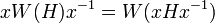# Conjugacy functor

This article defines a particular kind of map (functor) from a set of subgroups of a group to a set (possibly the same set) of subgroups

## History

### Origin of the term

The term was first used in the paper Transfer and fusion in finite groups by Alperin and Gorenstein in the Journal of Algebra, 6 (1967), Pages 242-255.

## Definition

### Definition with symbols

Let$G$ be a group and$p$ a prime. A conjugacy functor is a map$W$ from the collection of nontrivial$p$-subgroups of$G$ to the collection of nontrivial$p$-subgroups of$G$ that satisfies:

• For any$p$-subgroup$H$,$W(H) \le H$.
• For any$p$-subgroup$H$, and any$x \in G$,$xW(H)x^{-1} = W(xHx^{-1})$.

### Examples

Examples of conjugacy functors include the identity mapping, the functors corresponding to different possible Thompson subgroups, and the ZJ-functor.

Note also that any central functor is a conjugacy functor. Also, every characteristic p-functor is a conjugacy functor.